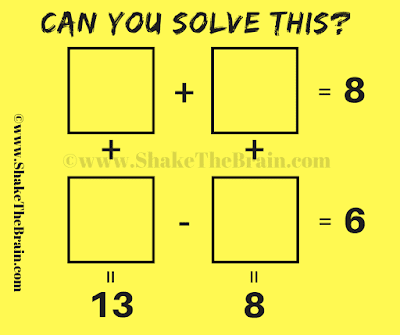This is a very interesting mathematical equation puzzle. Solving this math puzzle will help you to improve your mathematical skills. Here are four simultaneous equations and there are four variables. Can you solve these equations and write the correct answer in each box?Can you solve these Maths Equations?

The answer to this "Two Dimensional Mathematical Equations", can be viewed by clicking on the answer button.